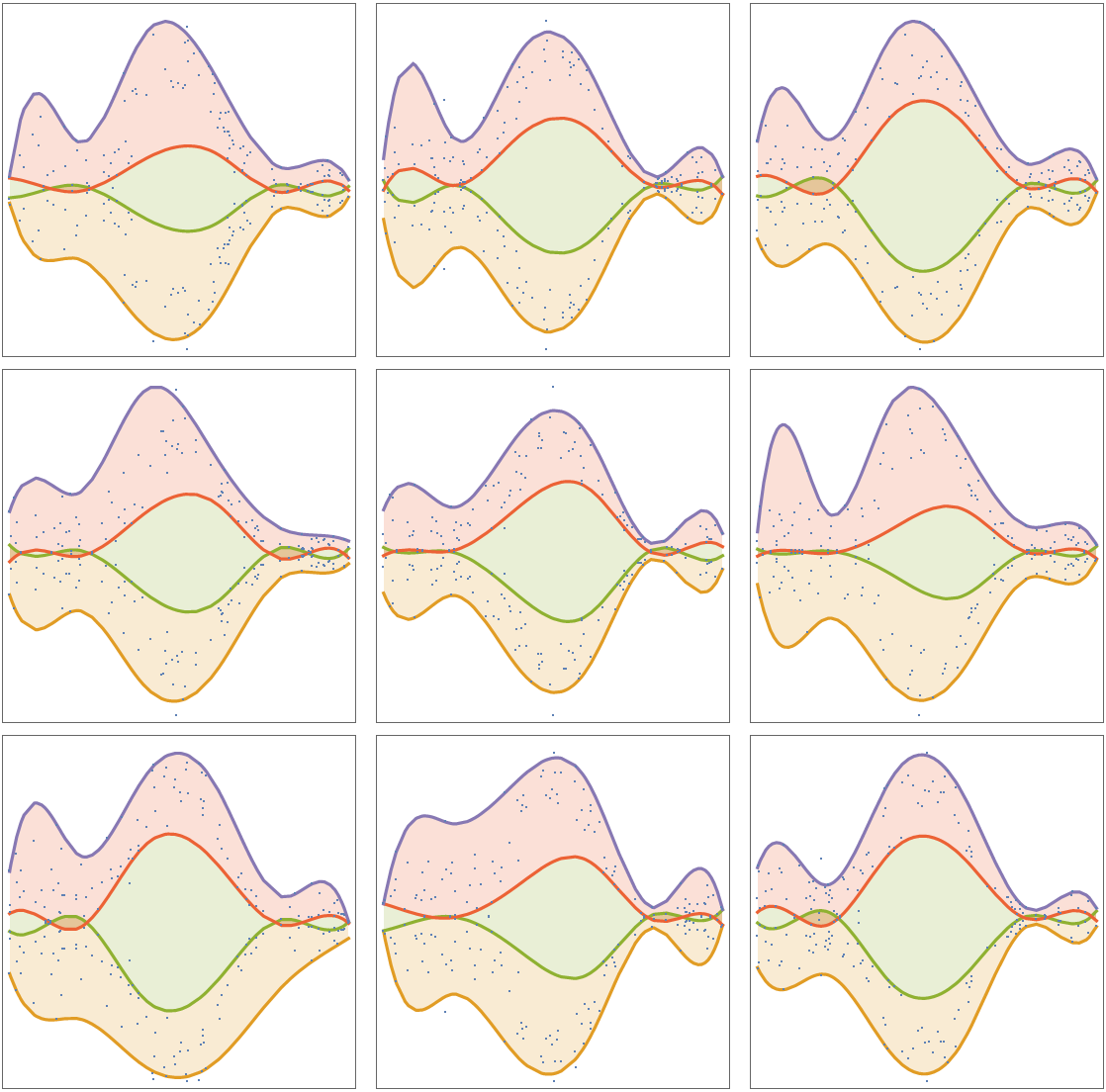#Function Repository Resource:

# QuantileRegression

Computes quantile regression fits over a time series, a list of numbers or a list of numeric pairs

Contributed by: Anton Antonov
 ResourceFunction["QuantileRegression"][data,knots,probs] does quantile regression over the times series or data array data using the knots specification knots for the probabilties probs. ResourceFunction["QuantileRegression"][data,knots,probs,opts] does quantile regression with the options opts.

## Details and Options

QuantileRegression works on time series, lists of numbers and lists of numeric pairs.
The curves computed with quantile regression are called regression quantiles.
The regression quantiles corresponding to the specified probabilities are linear combinations of B-splines generated over the specified knots.
In other words, QuantileRegression computes fits using a B-spline functions basis. The basis is specified with the knots argument and the option InterpolationOrder .
QuantileRegression takes the following options:
 3 interpolation order method for the quantile regression computations

## Examples

### Basic Examples

Make a random signal:

 In:=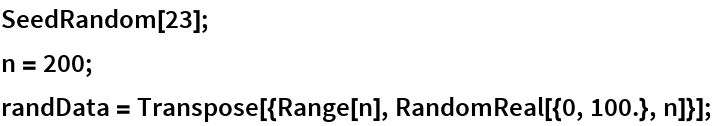Compute QuantileRegression with 5 knots for the probabilities 0.25 and 0.75:

 In:=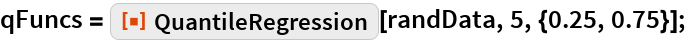Here are the formulas of the obtained regression quantiles:

 In:=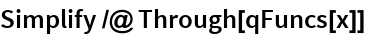Out=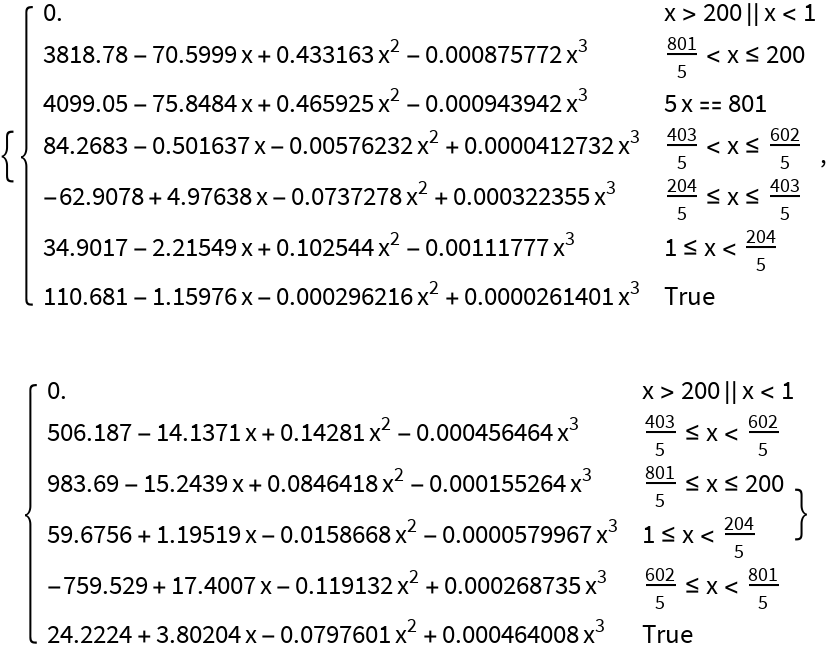Here is a plot of the original data and the obtained regression quantiles:

 In:=Out=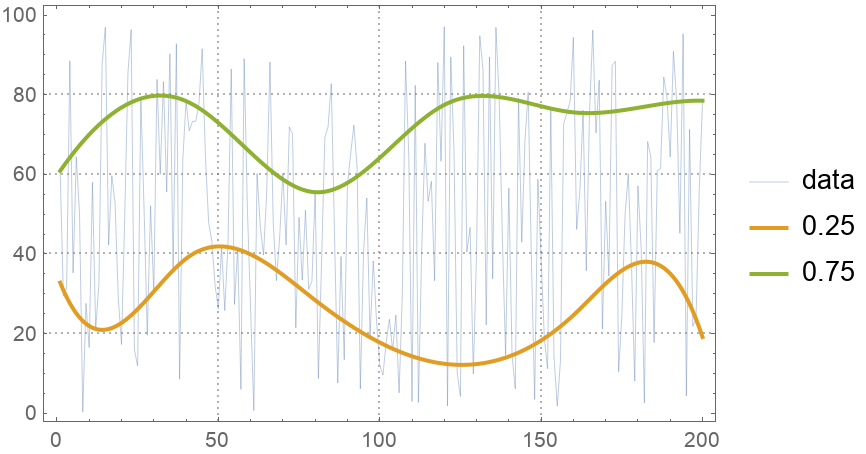Find the fraction of the data points that are under the second regression quantile:

 In:=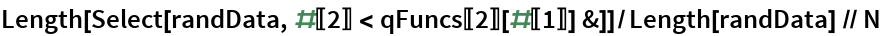Out=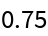The obtained fraction is close to the second probability, 0.75, given to QuantileRegression.

### Scope

Here is a quantile regression computation over a numerical vector:

 In:=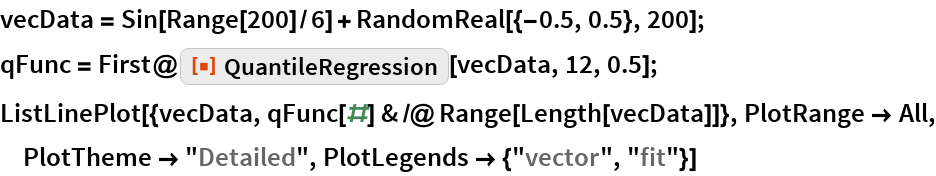Out=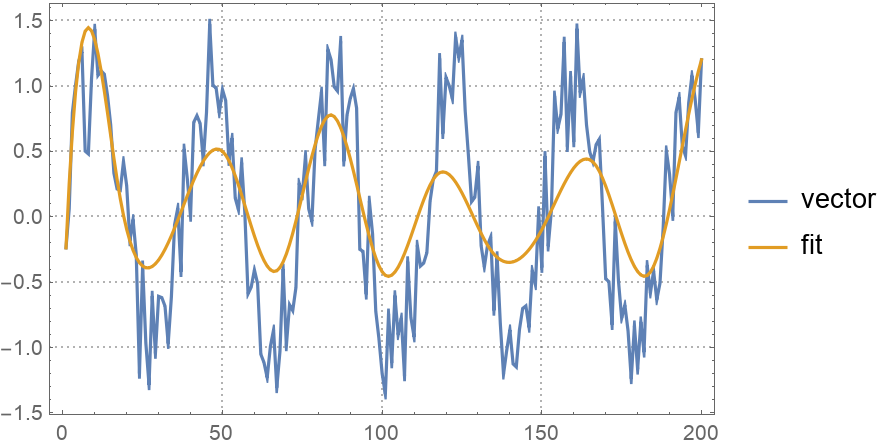Here is a quantile regression computation over a time series object:

 In:=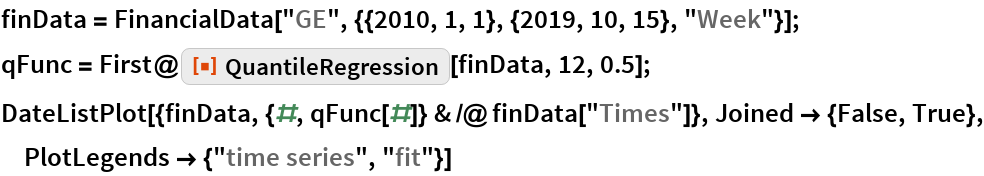Out=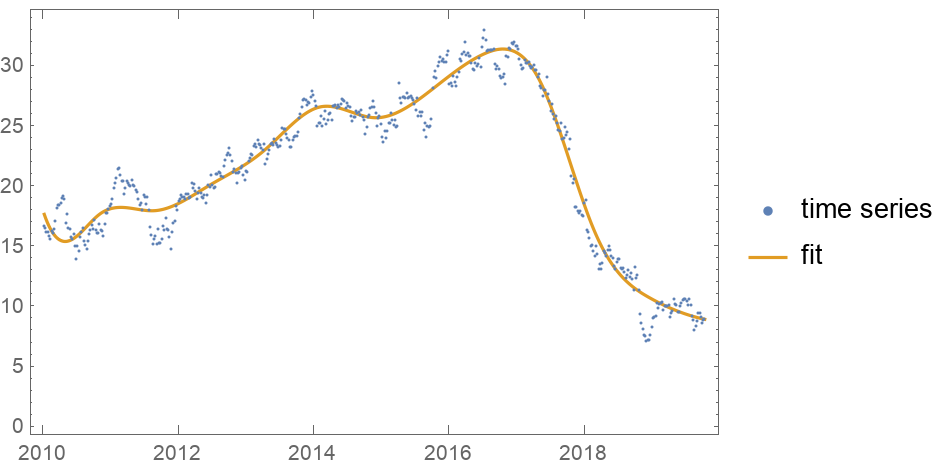The second argument—the knots specification—can be an integer specifying the number of knots or a list of numbers specifying the knots of the B-spline basis:

 In:=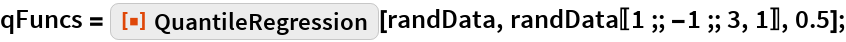### Options

#### InterpolationOrder

The option InterpolationOrder specifies the polynomial order of the B-spline basis. Its values are expected to be non-negative integers:

 In:=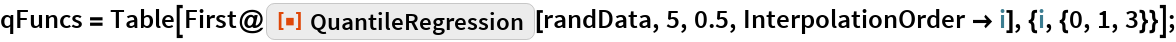In:=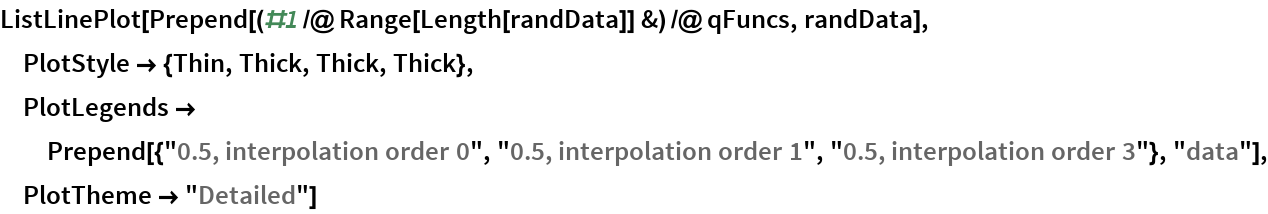Out=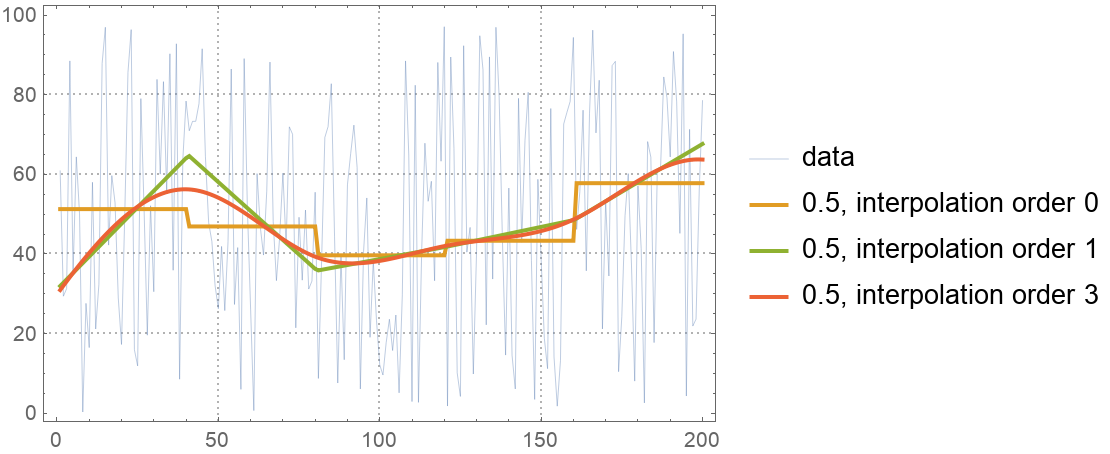#### Method

QuantileRegression uses LinearProgramming. Additional parameters can be passed to LinearProgramming with the Method option:

 In:=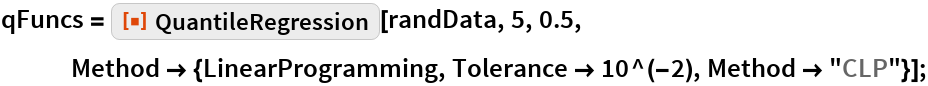In:=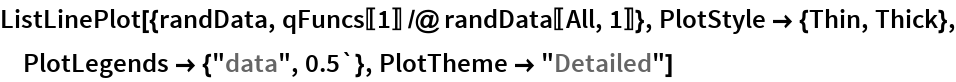Out=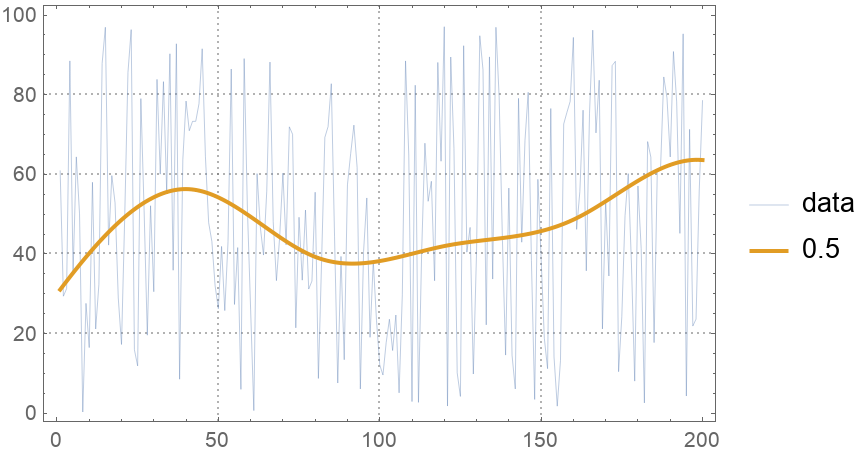### Applications

#### Fit for heteroscedastic data

Here is heteroscedastic data (the variance is not constant with respect to the predictor variable):

 In:=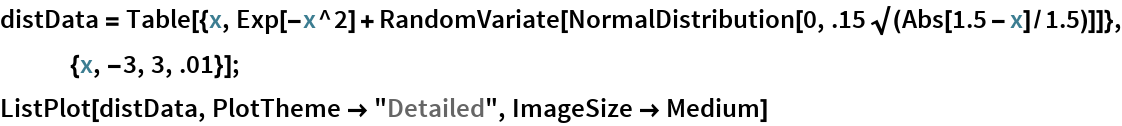Out=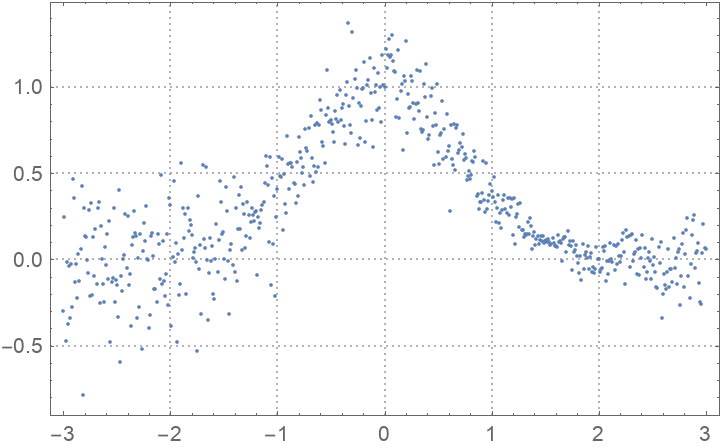Find quantile regression fits:

 In:=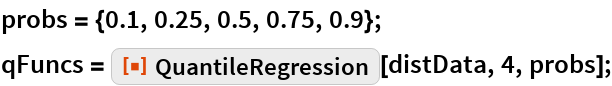Plot the data and the regression quantiles:

 In:=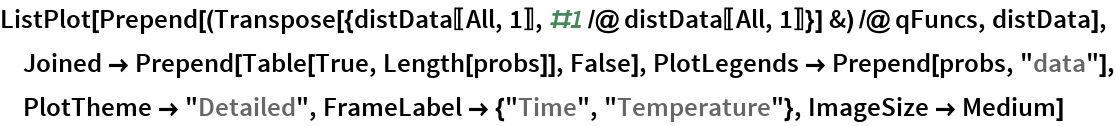Out=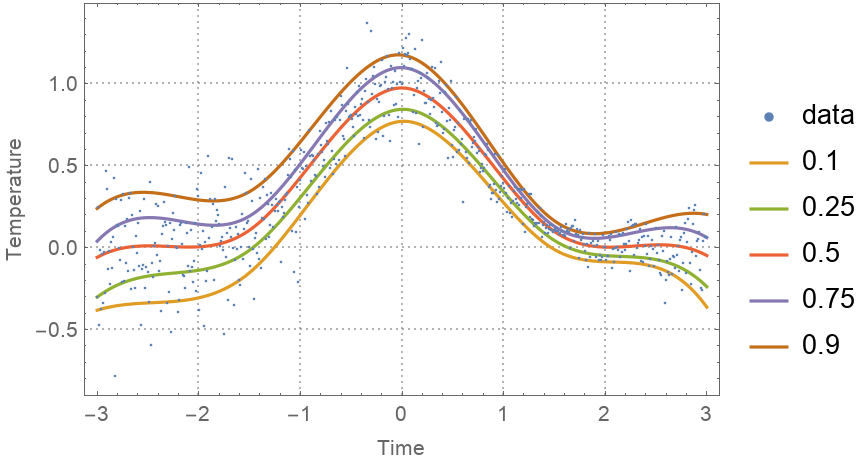Note that the regression quantiles clearly outline the heteroscedastic nature of the data.

#### Find variance anomalies

A certain contextual type of anomaly is a subset of points that have variance very different than other subsets. Using quantile regression we can (1) evaluate the regressor-dependent variance for each point using the regression quantiles 0.25 and 0.75; and (2) find the points that have outlier variances.

Here we compute and plot the variance estimates for a signal:

 In:=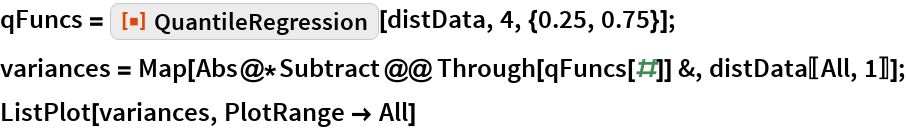Out=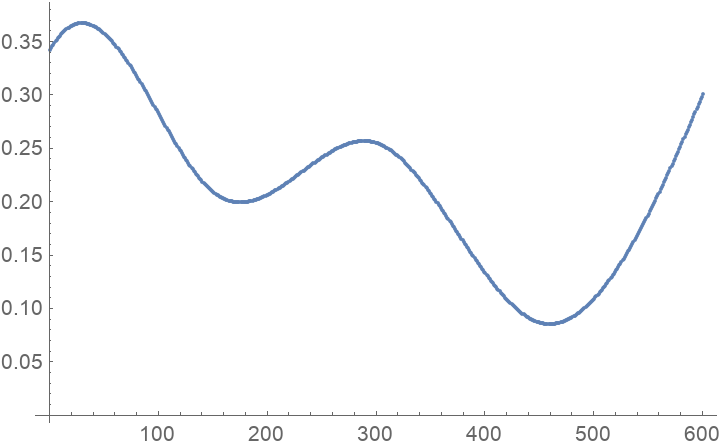Find the lower and upper thresholds for the variance outliers:

 In:=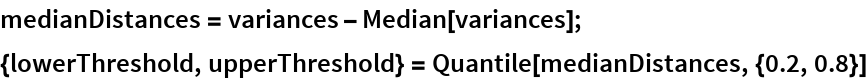Out=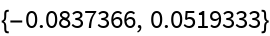Find the outlier positions:

 In:=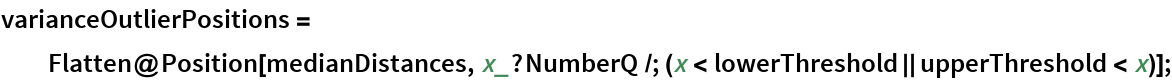Plot the data and the outliers found:

 In:=Out=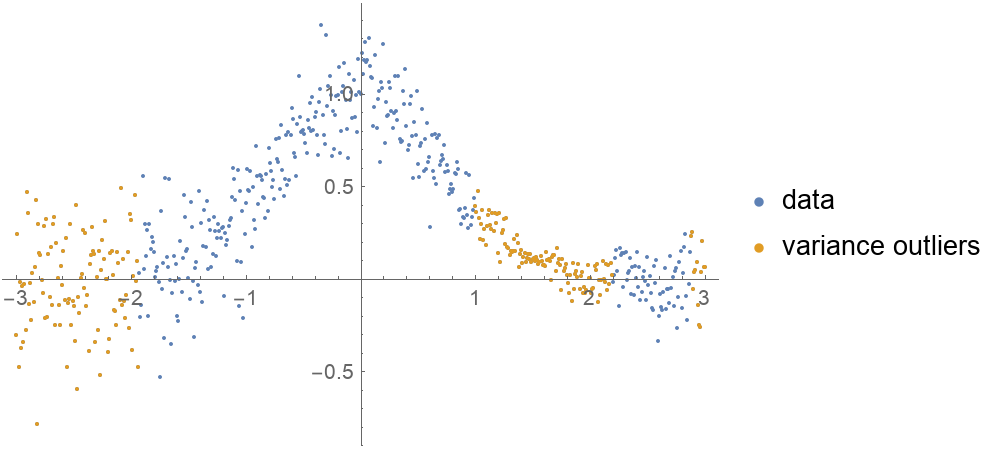#### Fit and anomalies for financial time series

Here is a financial time series:

 In:=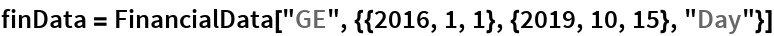Out=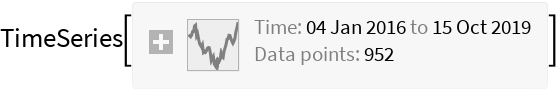In:=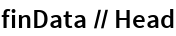Out=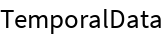Do a quantile regression fit and plot it:

 In:=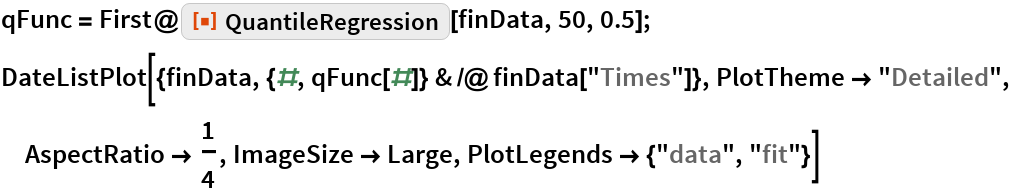Out=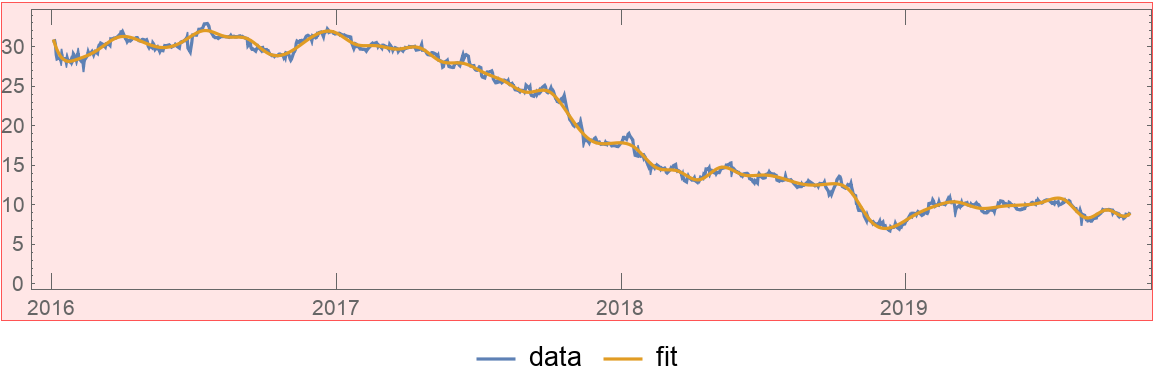Here are the errors of the fit found:

 In:=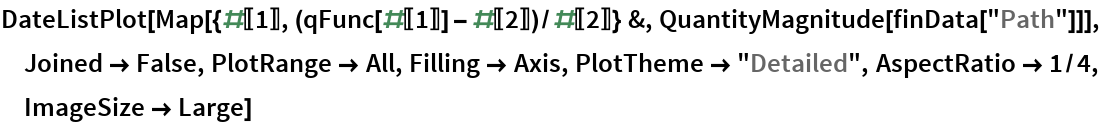Out=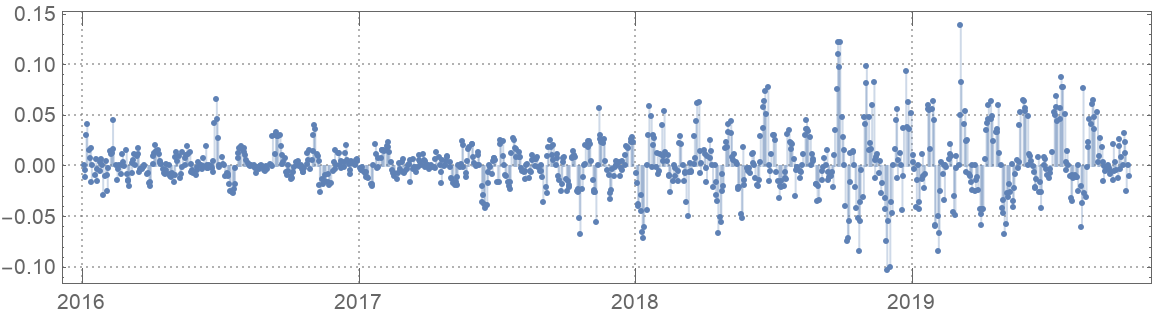Find anomalies’ positions in the list of fit errors:

 In:=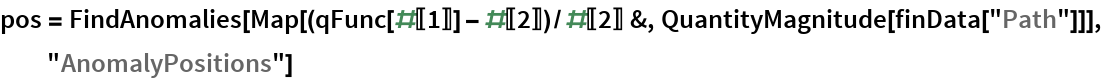Out=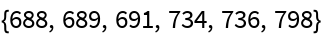Plot the data, fit and anomalies:

 In:=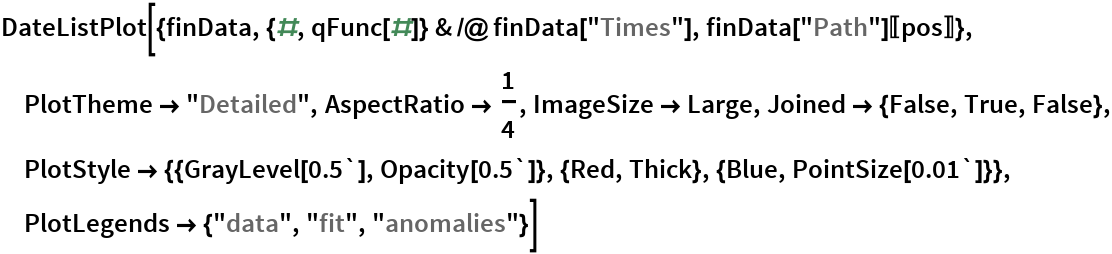Out=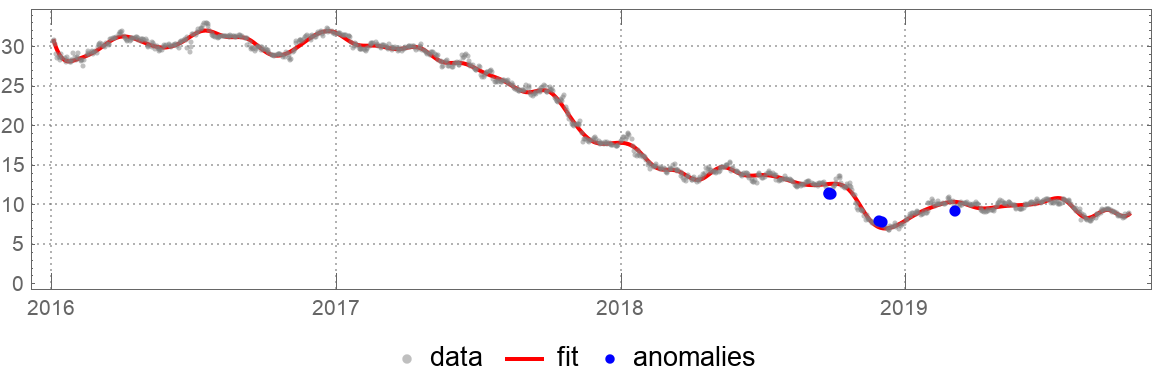#### Analyze temperature time series

Get temperature data:

 In:=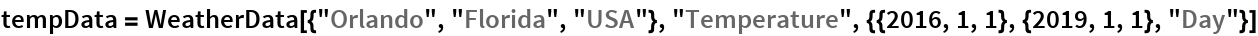Out=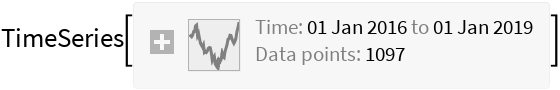Convert the time series into a list of numeric pairs:

 In:=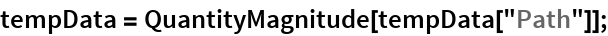Compute quantile regression fits:

 In:=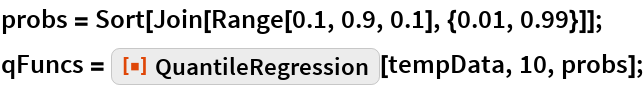Plot the data and the regression quantiles:

 In:=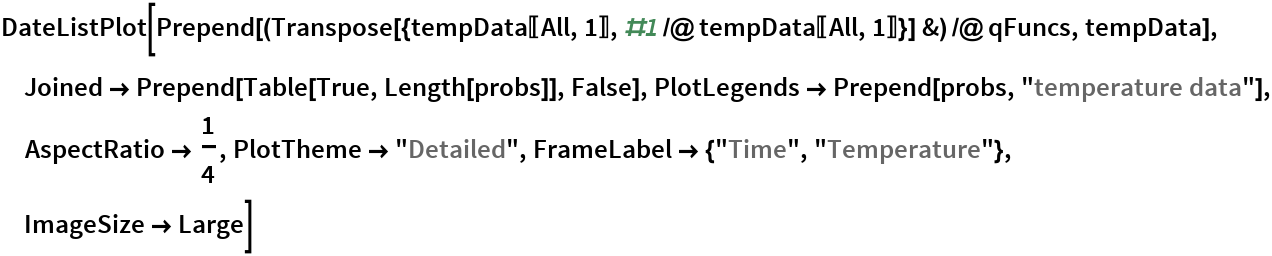Out=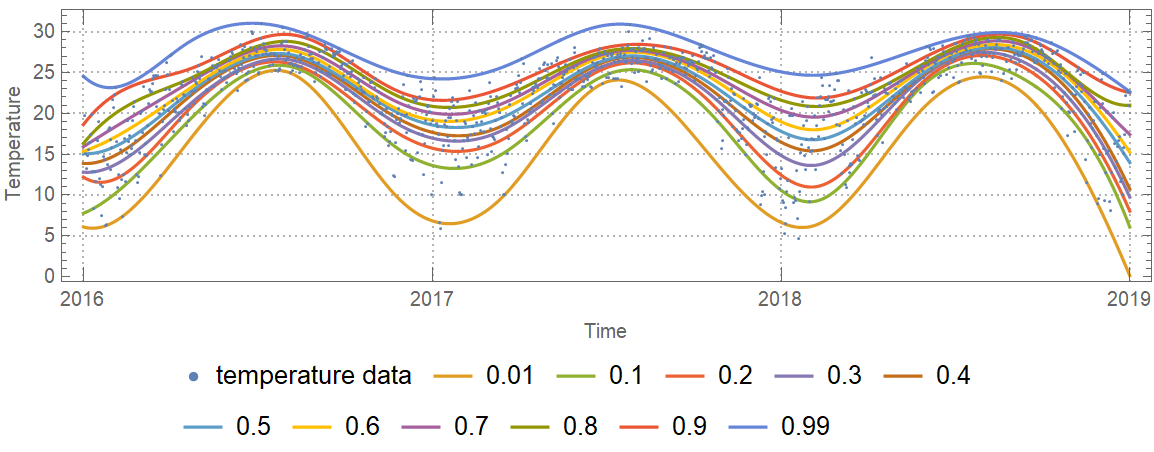Find an estimate of the conditional cumulative distribution function (CDF) at the date 2017-10-01:

 In:=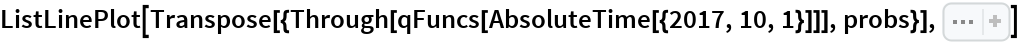Out=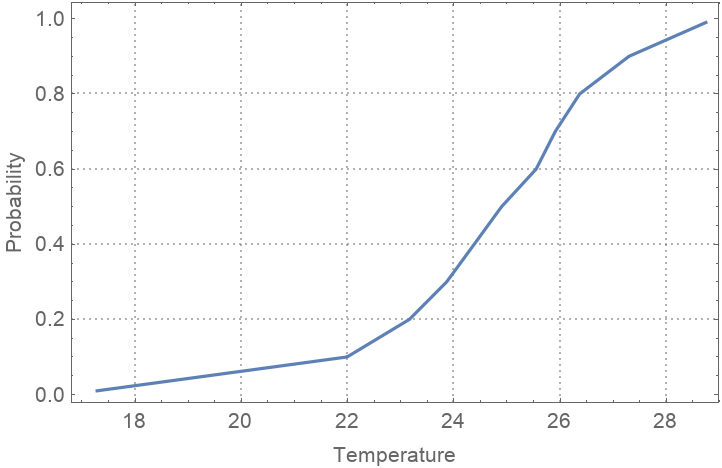Find outliers in the temperature data—outliers are defined as points below or above the 0.02 and 0.98 regression quantiles respectively:

 In:=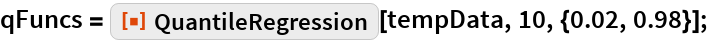In:=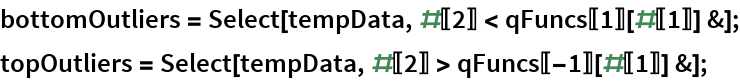In:=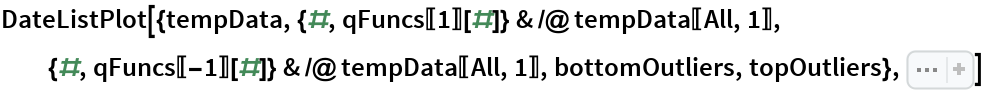Out=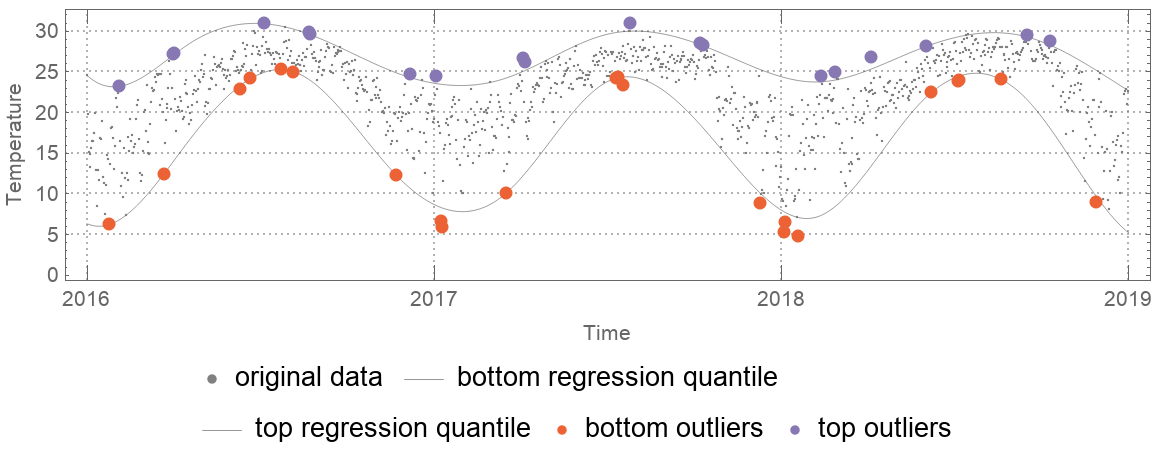### Properties and Relations

QuantileRegression can be compared with FindFormula, Fit , LinearModelFit and NonlinearModelFit:

 In:=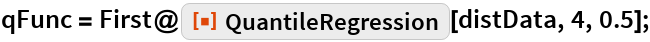In:=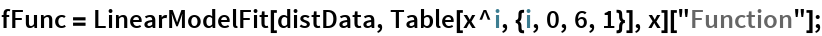In:=Out=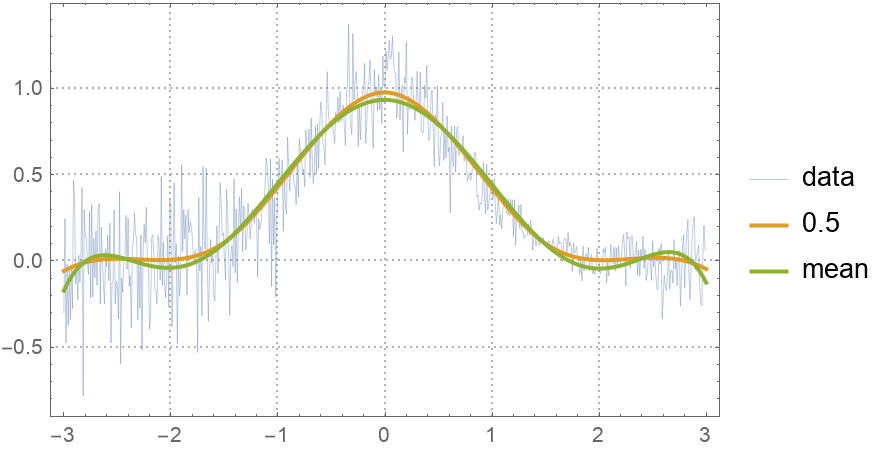Quantile regression is much more robust than linear regression. In order to demonstrate that, add a few large outliers in the data:

 In:=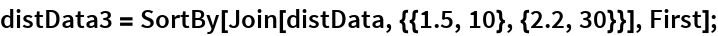Here quantile regression and linear regression are applied, as in the previous example:

 In:=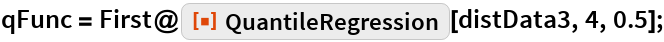In:=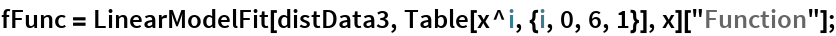Here is a plot of the obtained curves. Note that the curve corresponding to linear regression is different and a worse fit than the one from the previous example:

 In:=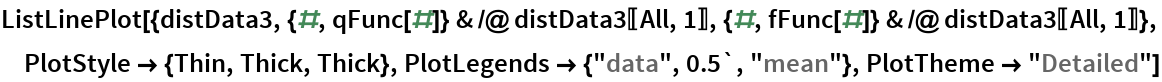Out=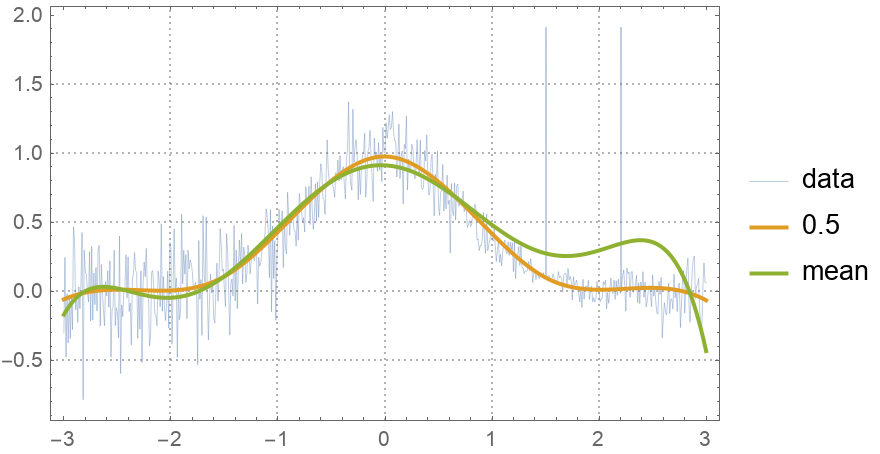### Possible Issues

#### Slow computations

Because of the linear programming formulation for some data and knots specifications, the computations can be slow.

#### Fitting for probabilities 0 and 1

For most data, the quantile regression fitting for probabilities 0 and 1 produces regression quantiles that are “too far away from the data.”

Find regression quantiles for probabilities 0 and 0.5 and plot them:

 In:=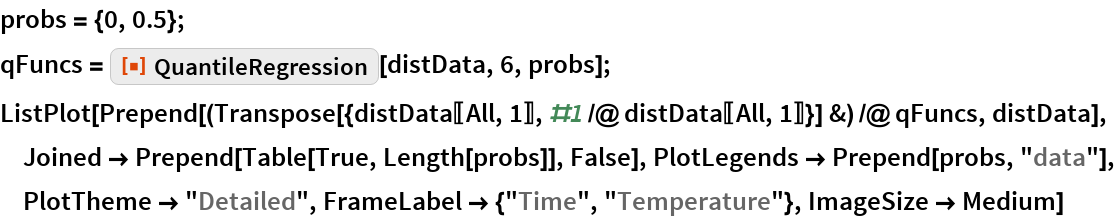Out=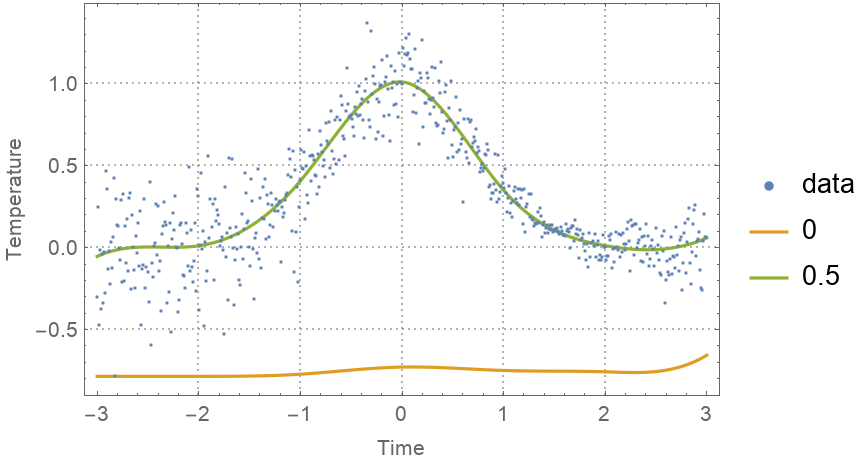Find regression quantiles for probabilities 0.5 and 1 and plot them:

 In:=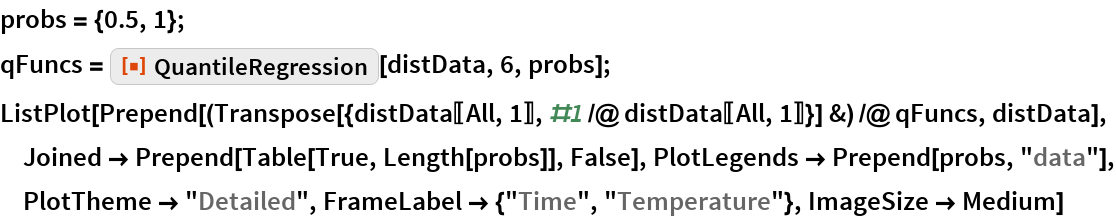Out=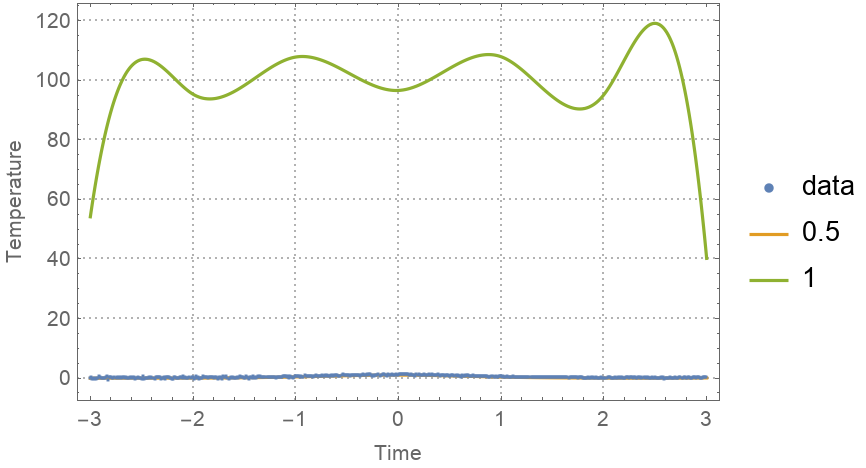One way to fix this is to use probabilities that are close to 0 and 1 from above and below, respectively:

 In:=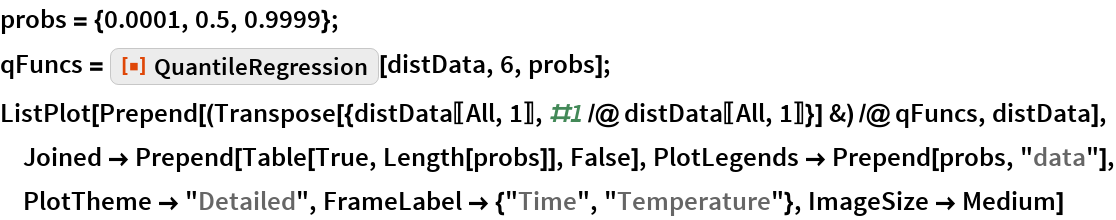Out=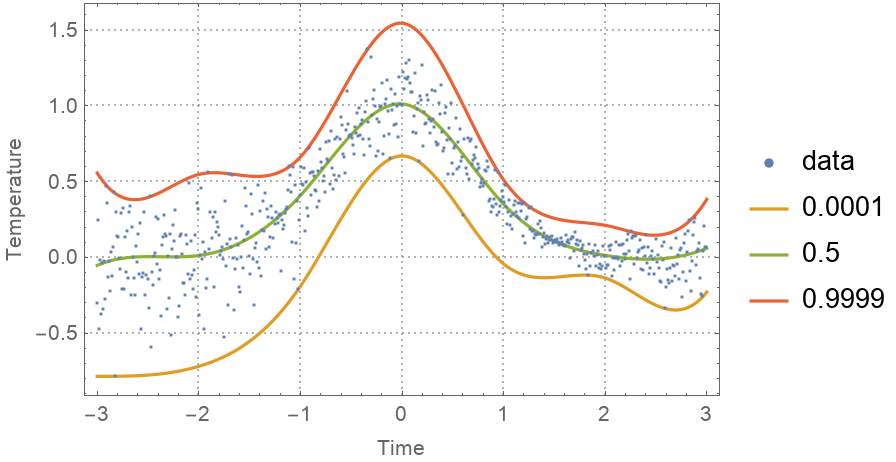#### Overfitting

Consider the following nonlinear data:

 In:=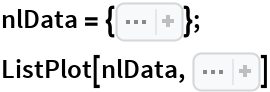Out=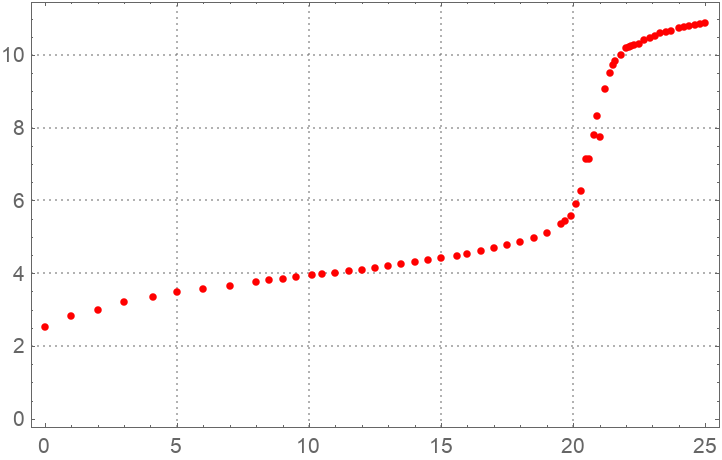Make a quantile regression fit with 20 knots:

 In:=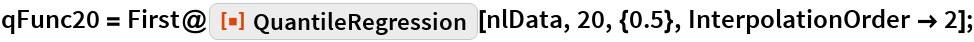Make a quantile regression fit with 40 knots:

 In:=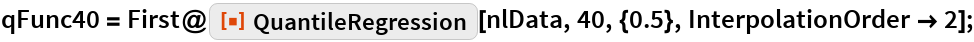Plot the regression quantiles and the data:

 In:=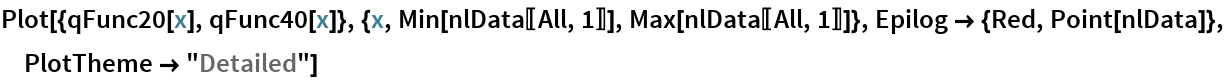Out=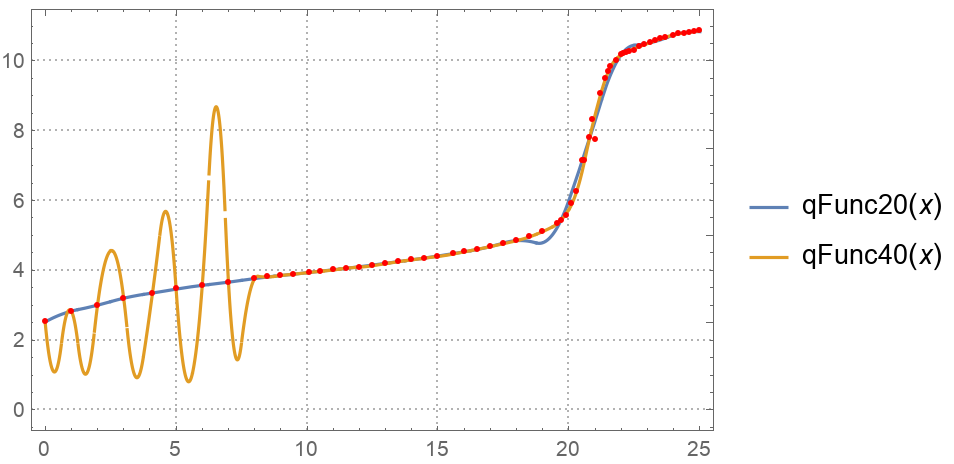You can see that the regression quantile computed with 40 knots is “overfitted” between 0 and 8—the B-spline basis knots are too densely placed between 0 and 8.

#### Intersecting regression quantiles

When regression quantiles are overfitted, then the estimate of the conditional cumulative distribution function (CDF) can be problematic—the estimated CDF is not a monotonically increasing function.

Compute regression quantiles using “too many” knots:

 In:=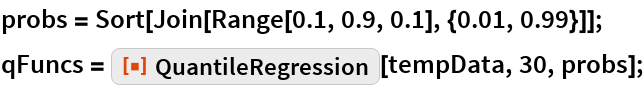Plot the regression quantiles:

 In:=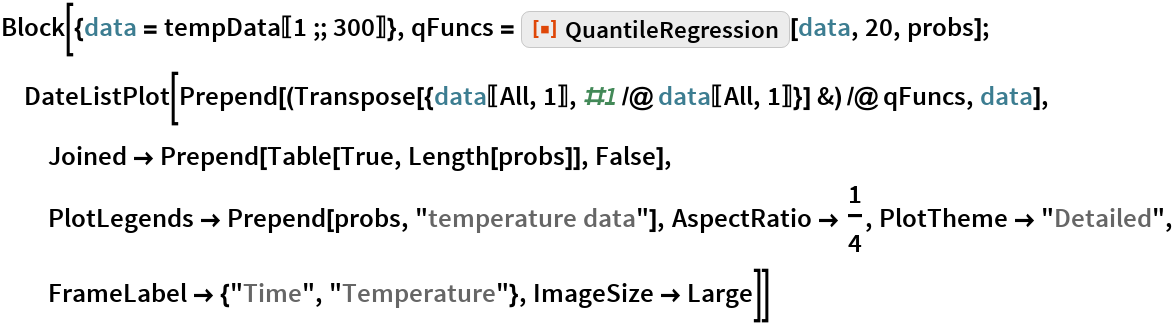Out=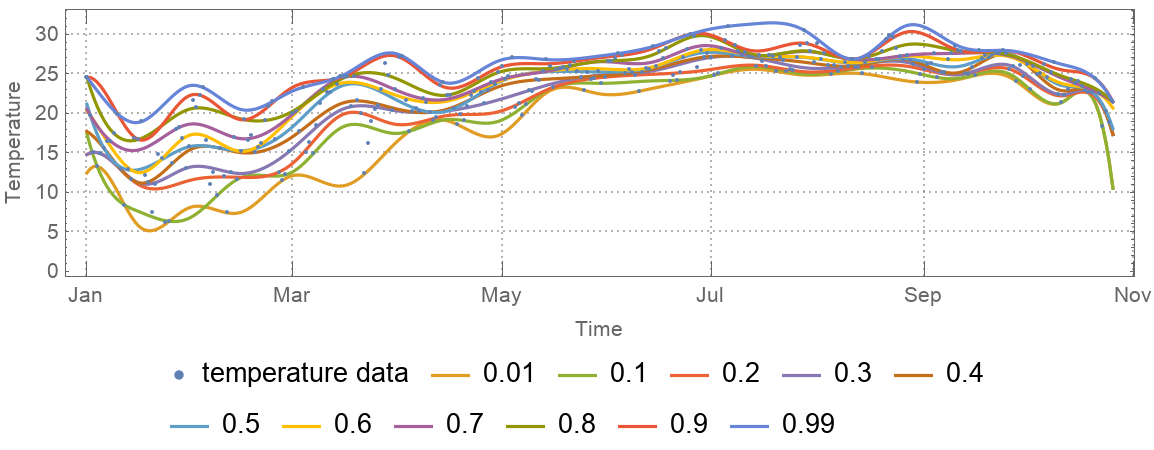Here is the estimated conditional CDF:

 In:=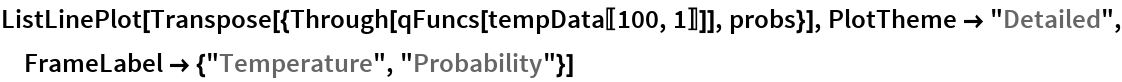Out=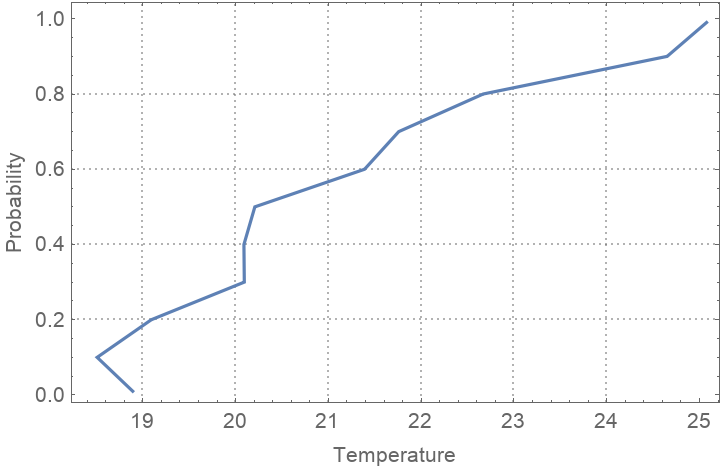#### Rescaling

For certain data, it is beneficial to rescale the predictor values, predicted values, or both before doing the quantile regression computations:

 In:=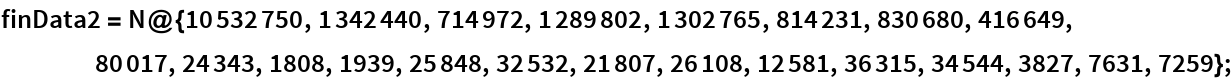In:=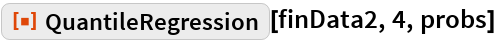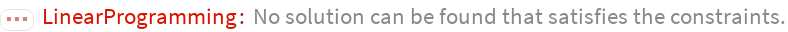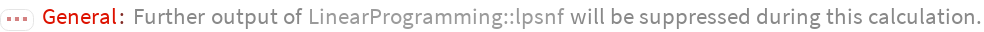Out=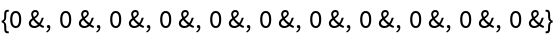In:=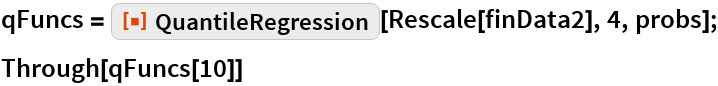Out=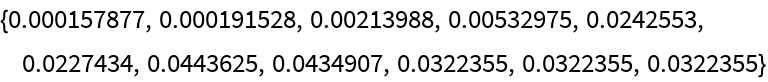### Neat Examples

Compute and plot regression quantiles over symmetric data:

 In:=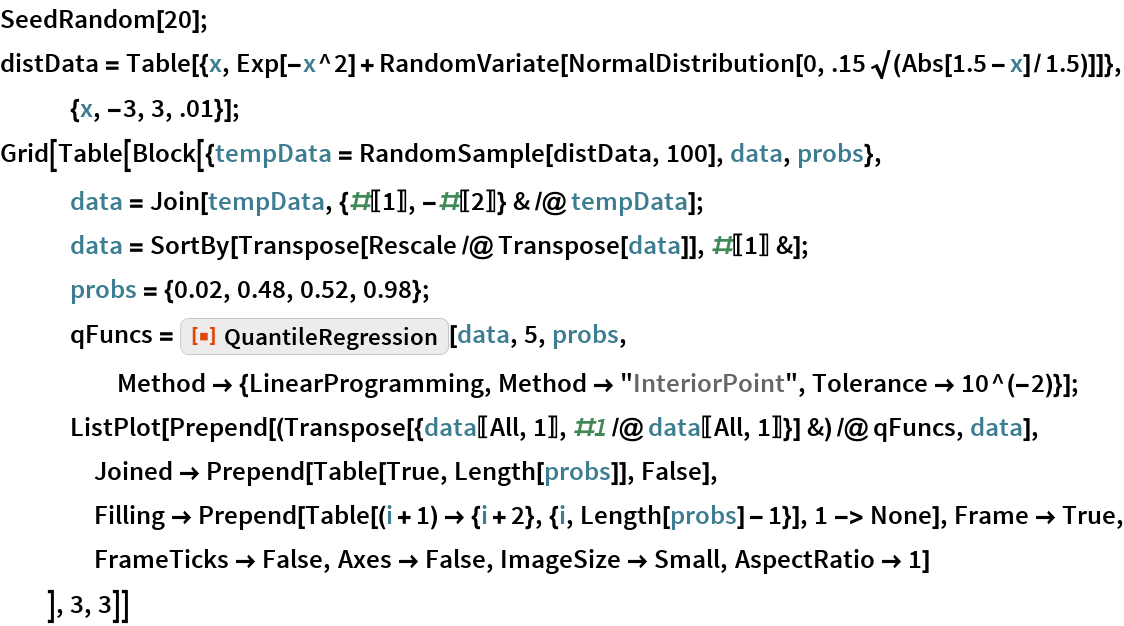Out=